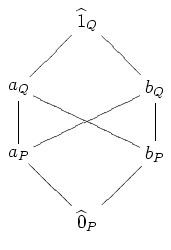# Star product

Updated on
Covid-19In mathematics, the star product is a method of combining graded posets with unique minimal and maximal elements, preserving the property that the posets are Eulerian.

## Definition

The star product of two graded posets ( P , P ) and ( Q , Q ) , where P has a unique maximal element 1 ^ and Q has a unique minimal element 0 ^ , is a poset P Q on the set ( P { 1 ^ } ) ( Q { 0 ^ } ) . We define the partial order P Q by x y if and only if:

1. { x , y } P , and x P y ; 2. { x , y } Q , and x Q y ; or 3. x P and y Q .

In other words, we pluck out the top of P and the bottom of Q , and require that everything in P be smaller than everything in Q .

## Example

For example, suppose P and Q are the Boolean algebra on two elements.

Then P Q is the poset with the Hasse diagram below.

## Properties

The star product of Eulerian posets is Eulerian.

## References

Similar Topics
Chaya Manush
John Child (volleyball)
Nikita Drozdov
Topics